# [handwritten numeral recognition] handwritten numeral recognition based on Matlab GUI knowledge base [including Matlab source code 1227]

2022-01-26 23:50:27

## One 、 Introduction to handwritten numeral recognition technology

1 Case background Handwritten numeral recognition is a branch of image recognition , It is one of the important applications in the field of image processing and pattern recognition , And it has strong universality . Due to the randomness of handwritten numbers , Such as stroke thickness 、 font size 、 Tilt angle and other factors may directly affect the accuracy of character recognition , So handwritten numeral recognition is a very challenging subject . In the past decades , Researchers have proposed many recognition methods , And achieved certain results . Handwritten numeral recognition is very practical , In large-scale data statistics, such as routine annual inspection 、 The census 、 financial 、 Taxation 、 Mail sorting and other application fields have broad application prospects ". This case describes the recognition process of handwritten Arabic numerals in images , The statistical method of handwritten numeral recognition is briefly introduced and analyzed , The experiment is carried out by developing a small handwritten numeral recognition system . Handwritten numeral recognition system needs to realize the reading function of handwritten numeral image 、 Feature extraction function 、 The establishment function and recognition function of digital template feature database .

2 Theoretical basis 2.1 Algorithm flow First , Read handwritten digital pictures for image normalization , Uniform size , The default is 24x24 Image block , And pass ostu The algorithm is binarized ; secondly , Carry out morphological operations such as image thinning on the binary image , And according to the algorithm requirements for feature extraction ; Last , Load the template matrix for comparison , Choose the Euclidean distance measure , Get recognition results . The algorithm flow chart is shown in the figure .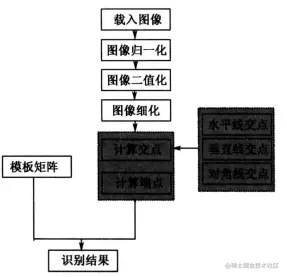2.2 feature extraction According to the structural characteristics of handwritten digital image itself , By calculating the endpoint 、 Specify the number of intersections of the direction line as the eigenvector . The main steps are as follows . （1） Vertical intersection For the thinned handwritten digital image, the column width of the thinned handwritten digital image is ：5/12 1/2 7/12 Generate three vertical lines at , Extract the intersection number of these three vertical lines and digital strokes and store . (2) Horizontal intersection For the thinned handwritten digital image, three horizontal lines are generated at the column width , Extract this 1/3 1/2 2/3 The number of intersections between the three horizontal lines and the number stroke and store . (3) Diagonal intersection Take two diagonal lines respectively for the thinned handwritten digital image , Extract the intersection number of these two diagonal lines and digital strokes and store . Since the above steps are applied to the thinned digital image , Its strokes are simple and its features are stable , Therefore, the extracted basic intersection and structural endpoint can reflect the essential characteristics of numbers , Fast 、 Effectively recognize numeric characters , And achieve better recognition accuracy . among , The algorithm for extracting stroke structure endpoint features is as follows . ① target location For the thinned handwritten digital image, press the line from top to bottom 、 Scan columns from left to right , Locate and select black pixels P As the target of handwriting stroke . ② Neighborhood statistics Calculate black pixels P Of 8 Sum of neighbors N, if N=1, Then pixels P For the endpoint , The endpoint counter is incremented 1; Otherwise, abandon the point . ③ Traversal image Traverse the entire image , Repeat target location 、 Operation flow of neighborhood statistics , Extract endpoint features . According to the intersection of handwritten digital image 、 Endpoint feature extraction method , The eigenvector in this case VEC from 9 It's made up of two components , They are arranged as follows ： VEC=[ vertical 5/12 Intersection points , The number of intersection points of vertical midline , vertical 7/12 Intersection points , level 1/3 Intersection points , Number of horizontal midline intersections , level 2/3 Intersection points , Left diagonal intersection points , Right diagonal intersection points , End points ]

2.3 pattern recognition This case adopts the recognition method based on pattern knowledge base , Therefore, the key step of systematic research is the analysis of the structural characteristics of digital characters and the construction of their models . therefore , This case first analyzes 09 this 10 The structure of a number character is analyzed and modeled , Then extract relevant features , Finally, construct the template library . During the experiment , We choose standard handwriting and free handwriting as training samples to adjust the parameters of the knowledge base , These training samples are made up of 200 A sample of standard handwriting and 200 A sample of free handwriting , Each component of the feature vector in the knowledge base is obtained by calculating the arithmetic mean of the corresponding components of the sample . The knowledge base obtained through the above steps is composed of two sets of templates , During this experiment , We choose the recognition method based on template matching , Measure the degree of matching by calculating the Euclidean distance . The feature vector in the recognition system contains 9 Weight , And the formula for calculating the distance is Euclidean distance ：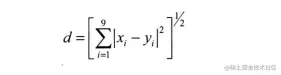therefore , In the recognition process, the Euclidean distance between the image to be recognized and each template feature vector in the knowledge base is calculated respectively , I.e 09 this 10 Compare the numbers one by one , Select the number corresponding to the minimum distance as the final recognition result .

## Two 、 Partial source code

``````function varargout = NumDemo(varargin)
% NUMDEMO MATLAB code for NumDemo.fig
%      NUMDEMO, by itself, creates a new NUMDEMO or raises the existing
%      singleton*.
%
%      H = NUMDEMO returns the handle to a new NUMDEMO or the handle to
%      the existing singleton*.
%
%      NUMDEMO('CALLBACK',hObject,eventData,handles,...) calls the local
%      function named CALLBACK in NUMDEMO.M with the given input arguments.
%
%      NUMDEMO('Property','Value',...) creates a new NUMDEMO or raises the
%      existing singleton*.  Starting from the left, property value pairs are
%      applied to the GUI before NumDemo_OpeningFcn gets called.  An
%      unrecognized property name or invalid value makes property application
%      stop.  All inputs are passed to NumDemo_OpeningFcn via varargin.
%
%      *See GUI Options on GUIDE's Tools menu.  Choose "GUI allows only one % instance to run (singleton)".
%

% Edit the above text to modify the response to help NumDemo

% Begin initialization code - DO NOT EDIT
gui_Singleton = 1;
gui_State = struct('gui_Name',       mfilename, ...
'gui_Singleton',  gui_Singleton, ...
'gui_OpeningFcn', @NumDemo_OpeningFcn, ...
'gui_OutputFcn',  @NumDemo_OutputFcn, ...
'gui_LayoutFcn',  [] , ...
'gui_Callback',   []);
if nargin && ischar(varargin{1}) gui_State.gui_Callback = str2func(varargin{1});
end

if nargout
[varargout{1:nargout}] = gui_mainfcn(gui_State, varargin{:});
else
gui_mainfcn(gui_State, varargin{:});
end
% End initialization code - DO NOT EDIT

% --- Executes just before NumDemo is made visible.
function NumDemo_OpeningFcn(hObject, ~, handles, varargin) % This function has no output args, see OutputFcn. % hObject handle to figure % eventdata reserved - to be defined in a future version of MATLAB % handles structure with handles and user data (see GUIDATA) % varargin command line arguments to NumDemo (see VARARGIN) % Choose default command line output for NumDemo handles.output = hObject;

% Update handles structure guidata(hObject, handles);

% UIWAIT makes NumDemo wait for user response (see UIRESUME) % uiwait(handles.figure1);

% --- Outputs from this function are returned to the command line.
function varargout = NumDemo_OutputFcn(~, ~, handles)
% varargout  cell array for returning output args (see VARARGOUT);
% hObject    handle to figure
% eventdata  reserved - to be defined in a future version of MATLAB
% handles structure with handles and user data (see GUIDATA) % Get default command line output from handles structure varargout{1} = handles.output;
axis([0 250 0 250]);
clc;

% --- Executes on mouse press over figure background, over a disabled or
% --- inactive control, or over an axes background.
function figure1_WindowButtonDownFcn(~, ~, handles) % hObject handle to figure1 (see GCBO) % eventdata reserved - to be defined in a future version of MATLAB % handles structure with handles and user data (see GUIDATA) global ButtonDown pos1 if strcmp(get(gcf, 'SelectionType'), 'normal') ButtonDown = 1;
pos1 = get(handles.axes1, 'CurrentPoint');
end

% --- Executes on mouse motion over figure - except title and menu.
function figure1_WindowButtonMotionFcn(~, ~, handles) % hObject handle to figure1 (see GCBO) % eventdata reserved - to be defined in a future version of MATLAB % handles structure with handles and user data (see GUIDATA) global ButtonDown pos1 if(ButtonDown == 1) pos = get(handles.axes1,'CurrentPoint');
line([pos1(1,1) pos(1,1)], [pos1(1,2) pos(1,2)], 'Color','Black','LineStyle','-', 'LineWidth',8,'Marker','.', 'MarkerSize',20);
pos1 = pos;
end

% --- Executes on mouse press over figure background, over a disabled or
% --- inactive control, or over an axes background.
function figure1_WindowButtonUpFcn(~, ~, ~) % hObject handle to figure1 (see GCBO) % eventdata reserved - to be defined in a future version of MATLAB % handles structure with handles and user data (see GUIDATA) global ButtonDown ButtonDown = 0;

% --- Executes on button press in pushbutton_clear.
function pushbutton_clear_Callback(~, ~, handles) % hObject handle to pushbutton_clear (see GCBO) % eventdata reserved - to be defined in a future version of MATLAB % handles structure with handles and user data (see GUIDATA) %cla;
cla(handles.axes1);

% --- Executes on button press in pushbutton_save.
function pushbutton_save_Callback(~, ~, handles) % hObject handle to pushbutton_save (see GCBO) % eventdata reserved - to be defined in a future version of MATLAB % handles structure with handles and user data (see GUIDATA) [f, p] = uiputfile({'*.bmp'},'Save Image');
str = strcat(p, f);
px  = getframe(handles.axes3);
curImg = frame2im(px);
imwrite(curImg, str,'bmp');

% --- Executes on button press in pushbutton_classify.
function pushbutton_classify_Callback(~, ~, handles) % hObject handle to pushbutton_classify (see GCBO) % eventdata reserved - to be defined in a future version of MATLAB % handles structure with handles and user data (see GUIDATA) %strSample = 'Data.mat';
cla(handles.axes4,handles.axes5,handles.axes6);
I1=handles.I1;
curFea = GetFeature(I1);
I2 = Normalize_Img(I1);
bw1 = Bw_Img(I1);
bw2 = Thin_Img(bw1);
bw = bw2;
sz = size(bw);
[r, c] = find(bw==1);
rect = [min(c) min(r) max(c)-min(c) max(r)-min(r)];
vs = rect(1)+rect(3)*[5/12 1/2 7/12];
hs = rect(2)+rect(4)*[1/3 1/2 2/3];
pt1 = [rect(1:2); rect(1:2)+rect(3:4)];
pt2 = [rect(1)+rect(3) rect(2); rect(1) rect(2)+rect(4)];
k1 = (pt1(1,2)-pt1(2,2)) / (pt1(1,1)-pt1(2,1));
x1 = 1:sz(2);
y1 = k1*(x1-pt1(1,1)) + pt1(1,2);
k2 = (pt2(1,2)-pt2(2,2)) / (pt2(1,1)-pt2(2,1));
x2 = 1:sz(2);
y2 = k2*(x2-pt2(1,1)) + pt2(1,2);
axes(handles.axes4);
imshow(I2,[]);
handles.I2 = I2;
hold on;
h = rectangle('Position', [rect(1:2)-1 rect(3:4)+2], 'EdgeColor', 'r', 'LineWidth', 2);
legend(h, ' Digital area marker ', 'Location', 'South');
axes(handles.axes5);
imshow(bw1,[]);
handles.bw1 = bw1;
axes(handles.axes6);
imshow(bw,[],'Border', 'Loose');
handles.bw = bw;
hold on;
h = [];
for i = 1 : length(hs)
h = [h plot([1 sz(2)], [hs(i) hs(i)], 'r-')];
end
for i = 1 : length(vs)
h = [h plot([vs(i) vs(i)], [1 sz(1)], 'g-')];
end
h = [h plot(x1, y1, 'y-')];
h = [h plot(x2, y2, 'm-')];
legend([h(1) h(4) h(7) h(8)], {' Level ', ' Vertical straight line ', ' Left diagonal ', ' Right diagonal '}, 'Location', 'BestOutside');
hold off;

%yLabel = euclid(curFea);
h = warndlg(num2str(yLabel),' Recognition result ');

% --- Executes on mouse press over axes background.
function axes1_ButtonDownFcn(hObject, eventdata, handles) % hObject handle to axes1 (see GCBO) % eventdata reserved - to be defined in a future version of MATLAB % handles structure with handles and user data (see GUIDATA) % --- If Enable == 'on', executes on mouse press in 5 pixel border.
% --- Otherwise, executes on mouse press in 5 pixel border or over pushbutton_clear.
function pushbutton_clear_ButtonDownFcn(hObject, eventdata, handles)
% hObject    handle to pushbutton_clear (see GCBO)
% eventdata  reserved - to be defined in a future version of MATLAB
% handles    structure with handles and user data (see GUIDATA)
function testsample = GetFeature(I)
clc;
testsample = zeros(1,9);  %1 That's ok 9 Columns of the matrix
% The extracted features
I1 = Normalize_Img(I);
bw1 = Bw_Img(I1);
bw2 = Thin_Img(bw1);
bw = bw2;
sz = size(bw);
[r, c] = find(bw==1);
rect = [min(c) min(r) max(c)-min(c) max(r)-min(r)];
vs = rect(1)+rect(3)*[5/12 1/2 7/12];
hs = rect(2)+rect(4)*[1/3 1/2 2/3];
pt1 = [rect(1:2); rect(1:2)+rect(3:4)];
pt2 = [rect(1)+rect(3) rect(2); rect(1) rect(2)+rect(4)];
k1 = (pt1(1,2)-pt1(2,2)) / (pt1(1,1)-pt1(2,1));
x1 = 1:sz(2);
y1 = k1*(x1-pt1(1,1)) + pt1(1,2);
k2 = (pt2(1,2)-pt2(2,2)) / (pt2(1,1)-pt2(2,1));
x2 = 1:sz(2);
y2 = k2*(x2-pt2(1,1)) + pt2(1,2);
if flag
% figure('Name', ' Number recognition ', 'NumberTitle', 'Off', 'Units', 'Normalized', 'Position', [0.2 0.45 0.5 0.3]);
%subplot(2, 2, 1); imshow(I1, []); title(' Original image ', 'FontWeight', 'Bold');
%subplot(2, 2, 2); imshow(I2, []); title(' Normalized image ', 'FontWeight', 'Bold');
axes(handles.axes4);
imshow(I2,[]); title(' Normalized image ', 'FontWeight', 'Bold');
handles.I2 = I2;
hold on;
h = rectangle('Position', [rect(1:2)-1 rect(3:4)+2], 'EdgeColor', 'r', 'LineWidth', 2);

%  subplot(2, 2, 3); imshow(bw1, []); title(' Binary image ', 'FontWeight', 'Bold');

%   subplot(2, 2, 4); imshow(bw, [], 'Border', 'Loose'); title(' Thinning image ', 'FontWeight', 'Bold');
hold on;
h = [];
for i = 1 : length(hs)
h = [h plot([1 sz(2)], [hs(i) hs(i)], 'r-')];
end
for i = 1 : length(vs)
h = [h plot([vs(i) vs(i)], [1 sz(1)], 'g-')];
end

hold off;
end
v{1} = [1:sz(2); repmat(hs(1), 1, sz(2))]'; v{2} = [1:sz(2); repmat(hs(2), 1, sz(2))]';
v{3} = [1:sz(2); repmat(hs(3), 1, sz(2))]'; v{4} = [repmat(vs(1), 1, sz(1)); 1:sz(1)]';
v{5} = [repmat(vs(2), 1, sz(1)); 1:sz(1)]'; v{6} = [repmat(vs(3), 1, sz(1)); 1:sz(1)]';
v{7} = [x1; y1]'; v{8} = [x2; y2]';

for i = 1 : 8
num(i) = GetImgLinePts(bw, round(v{i})-1);
end num(9) = sum(sum(endpoints(bw)));
testsample=num;
Copy code ``````

## 3、 ... and 、 Running results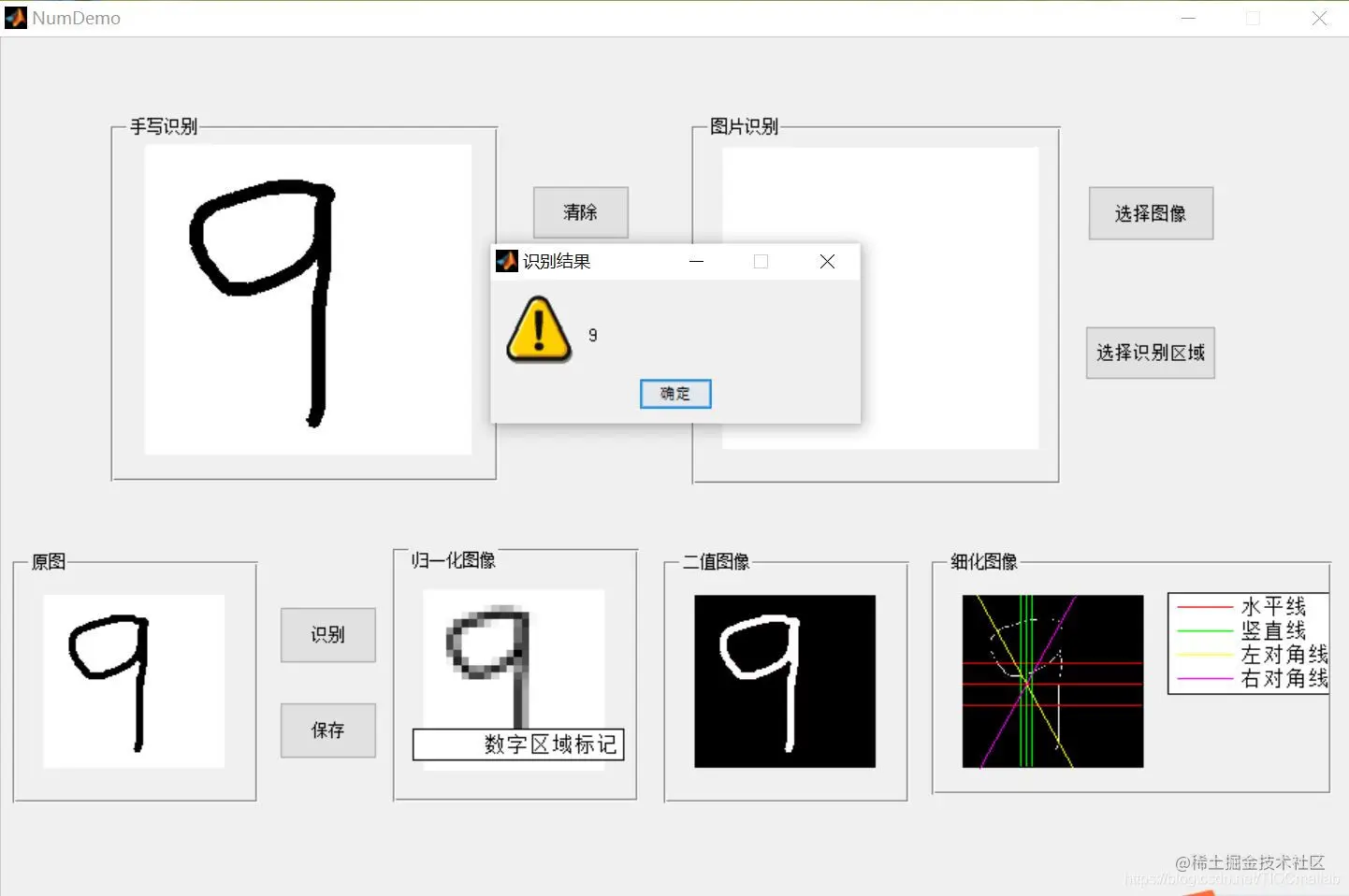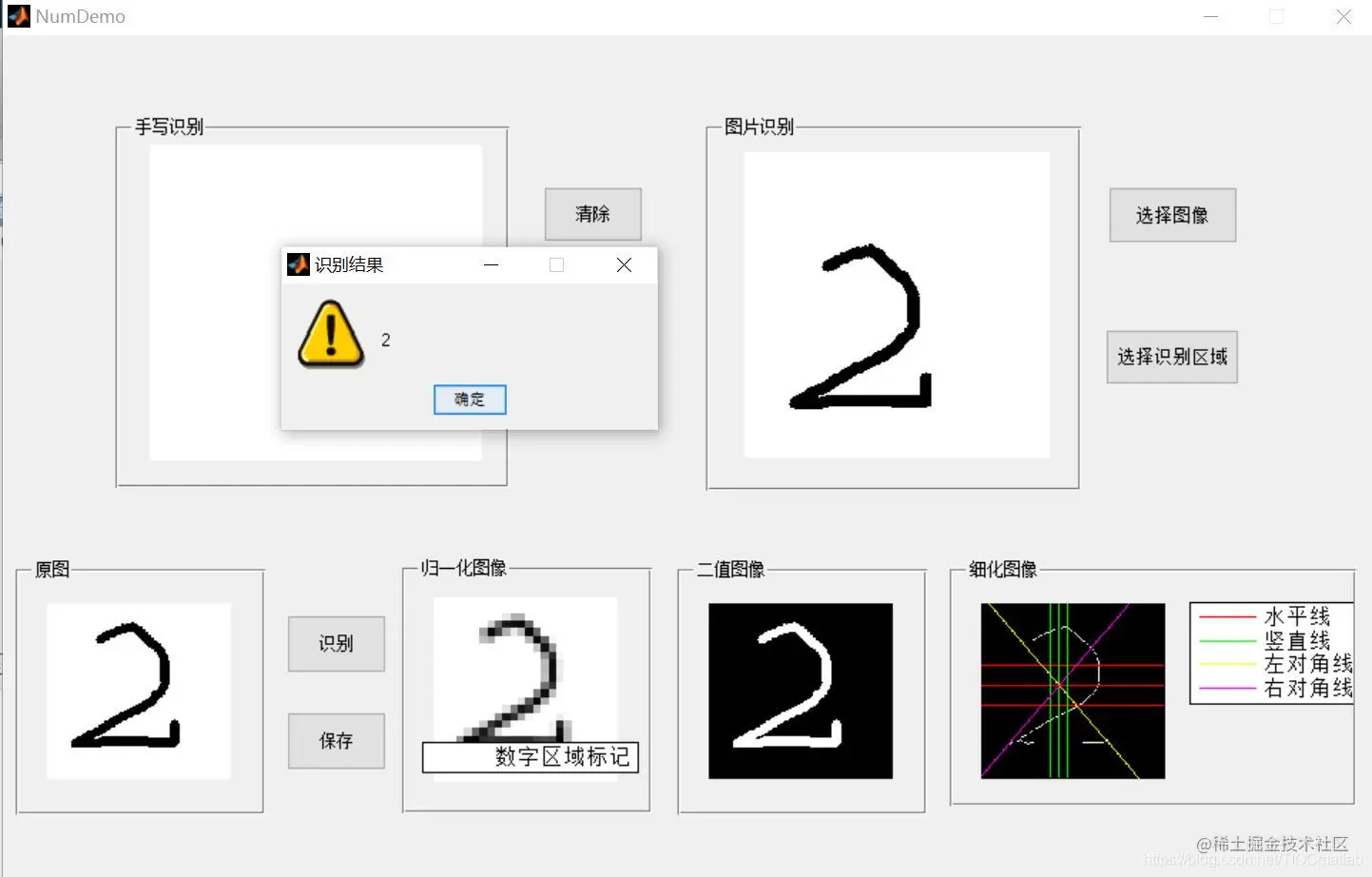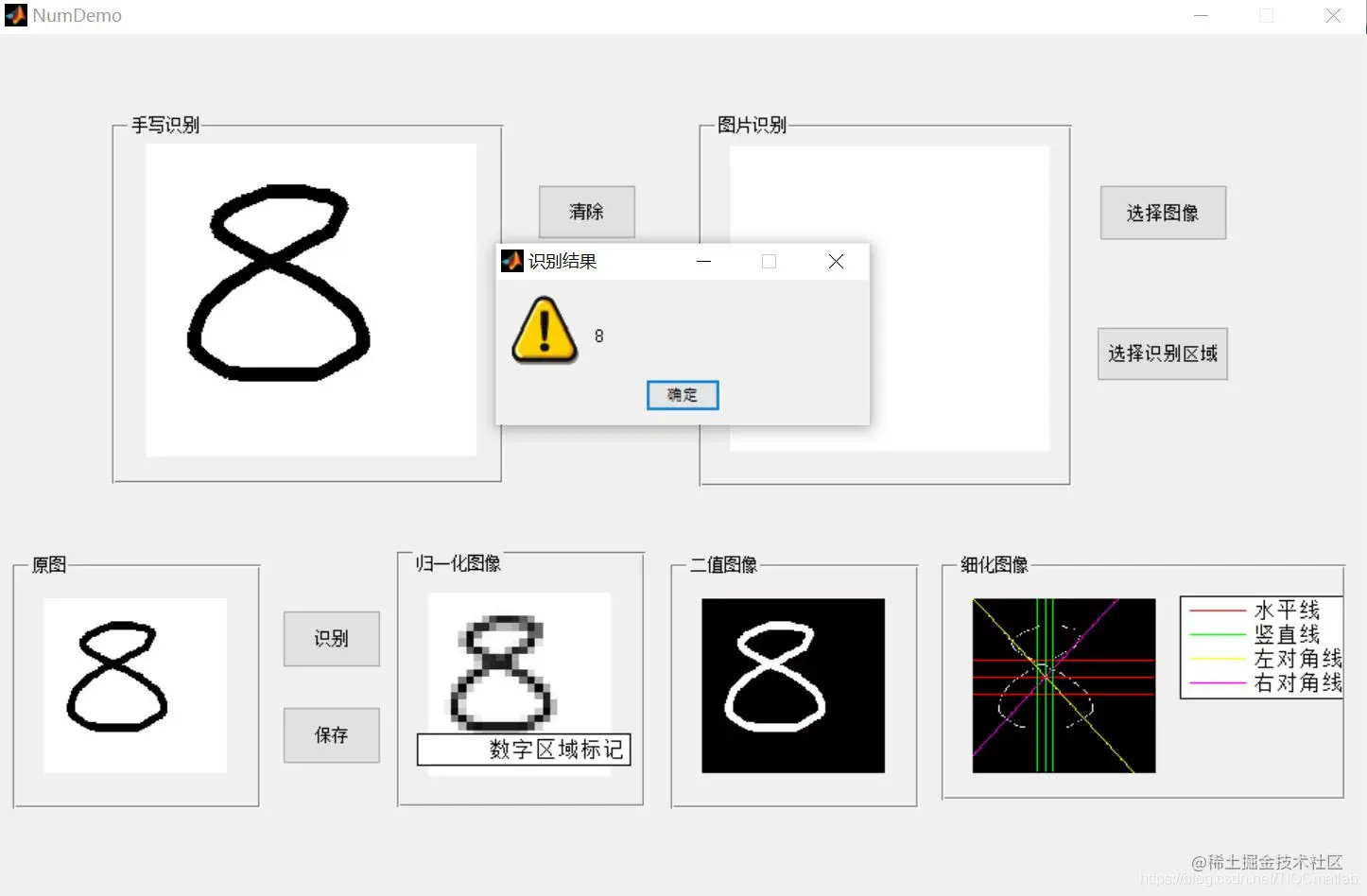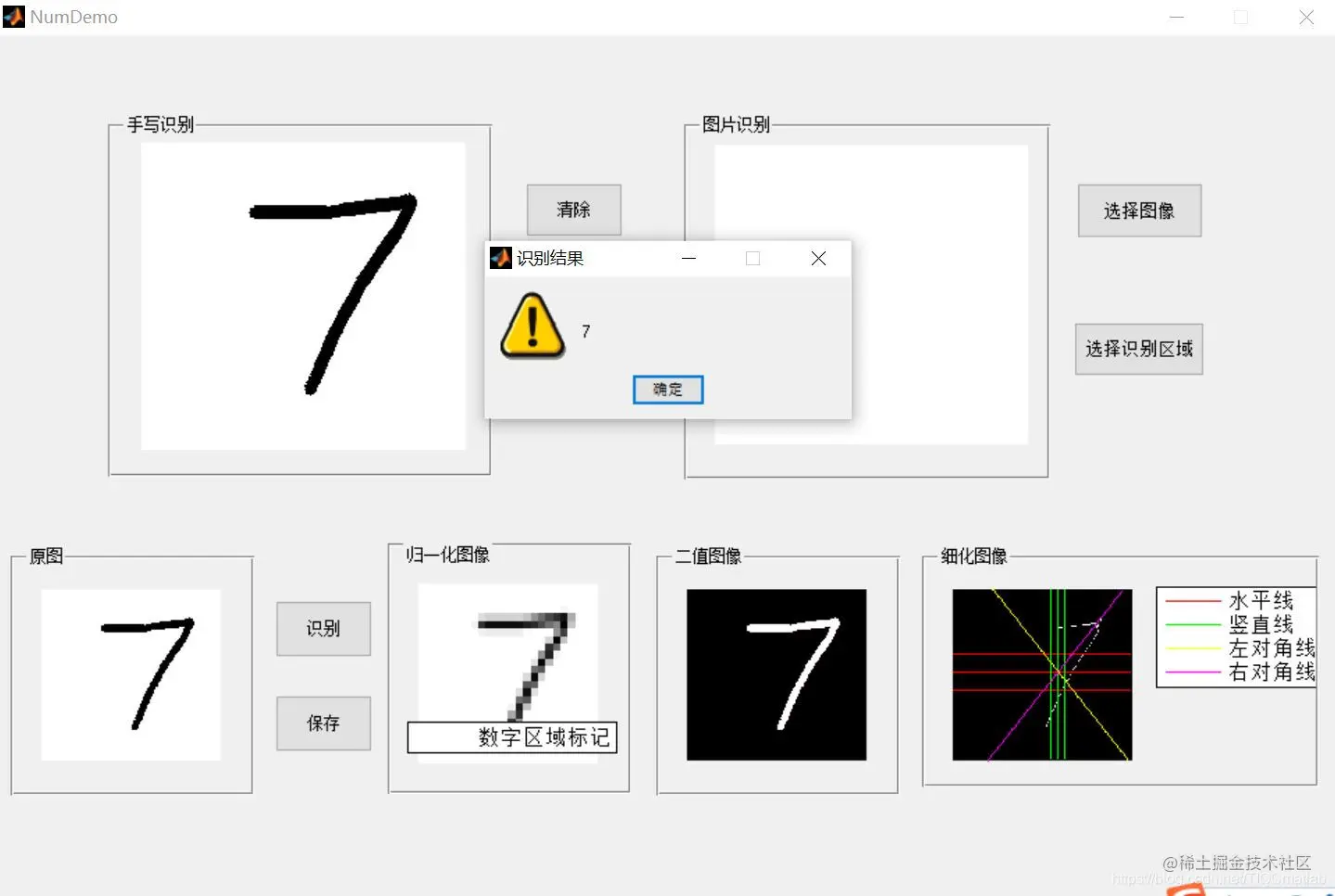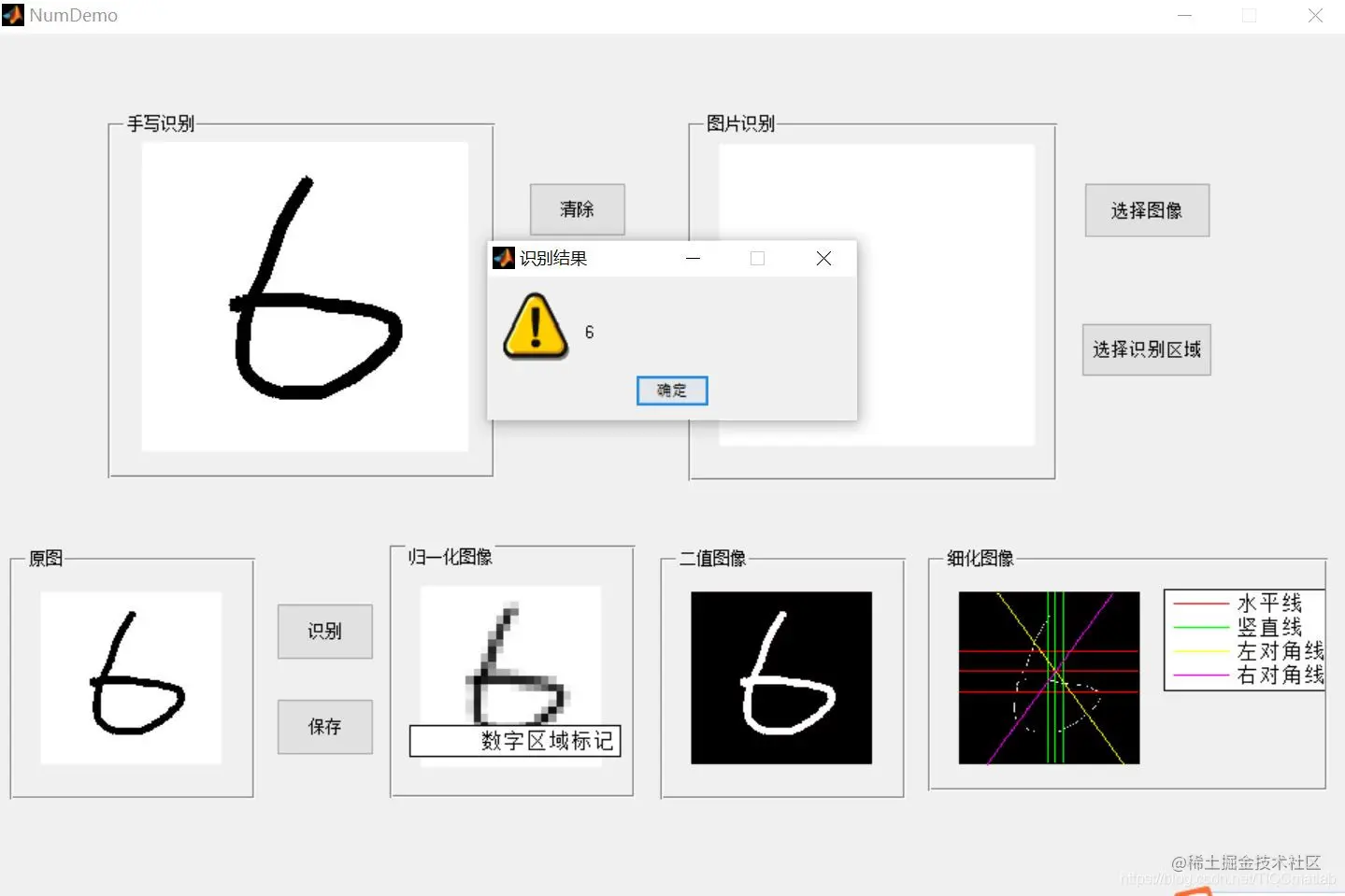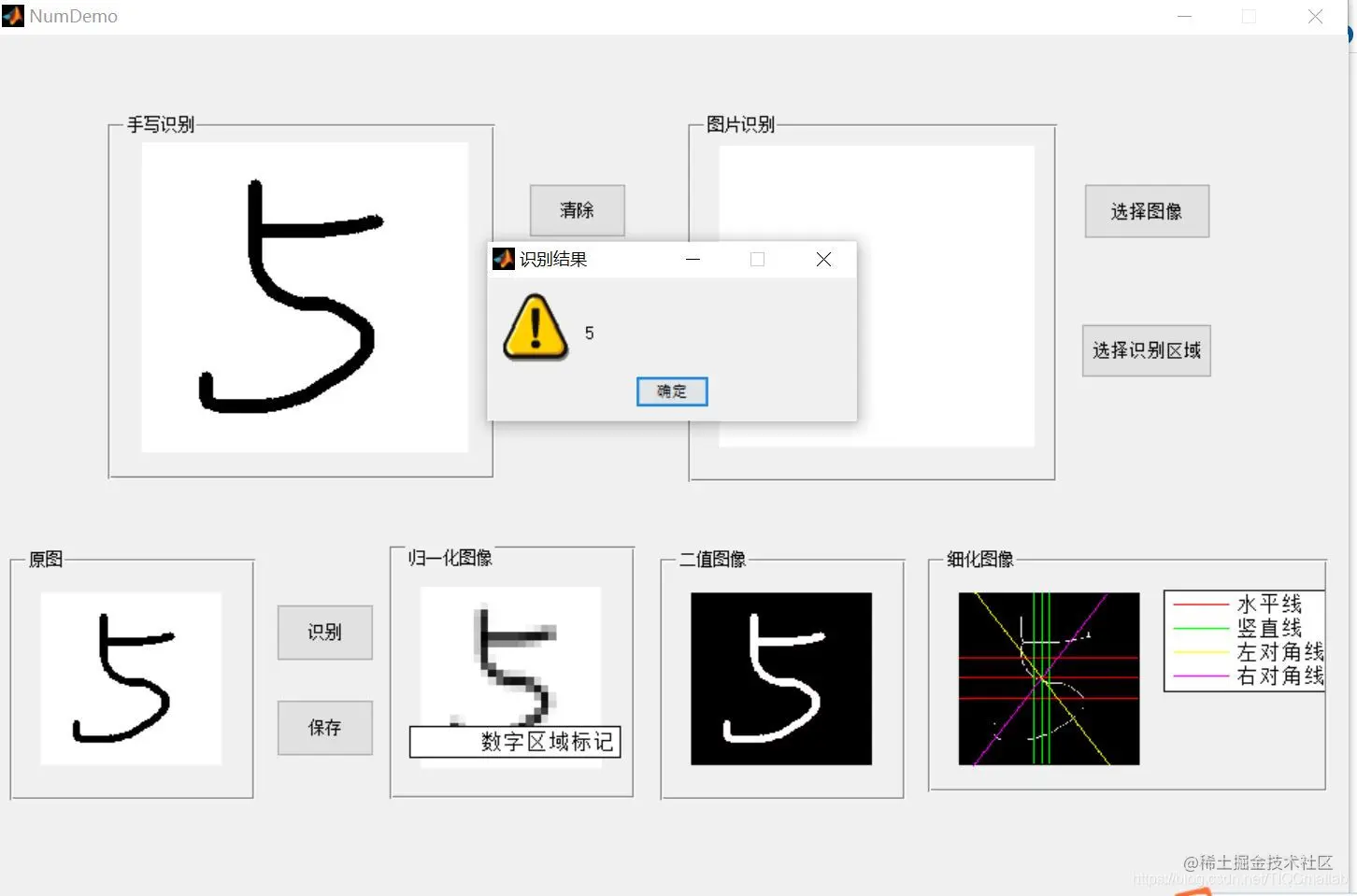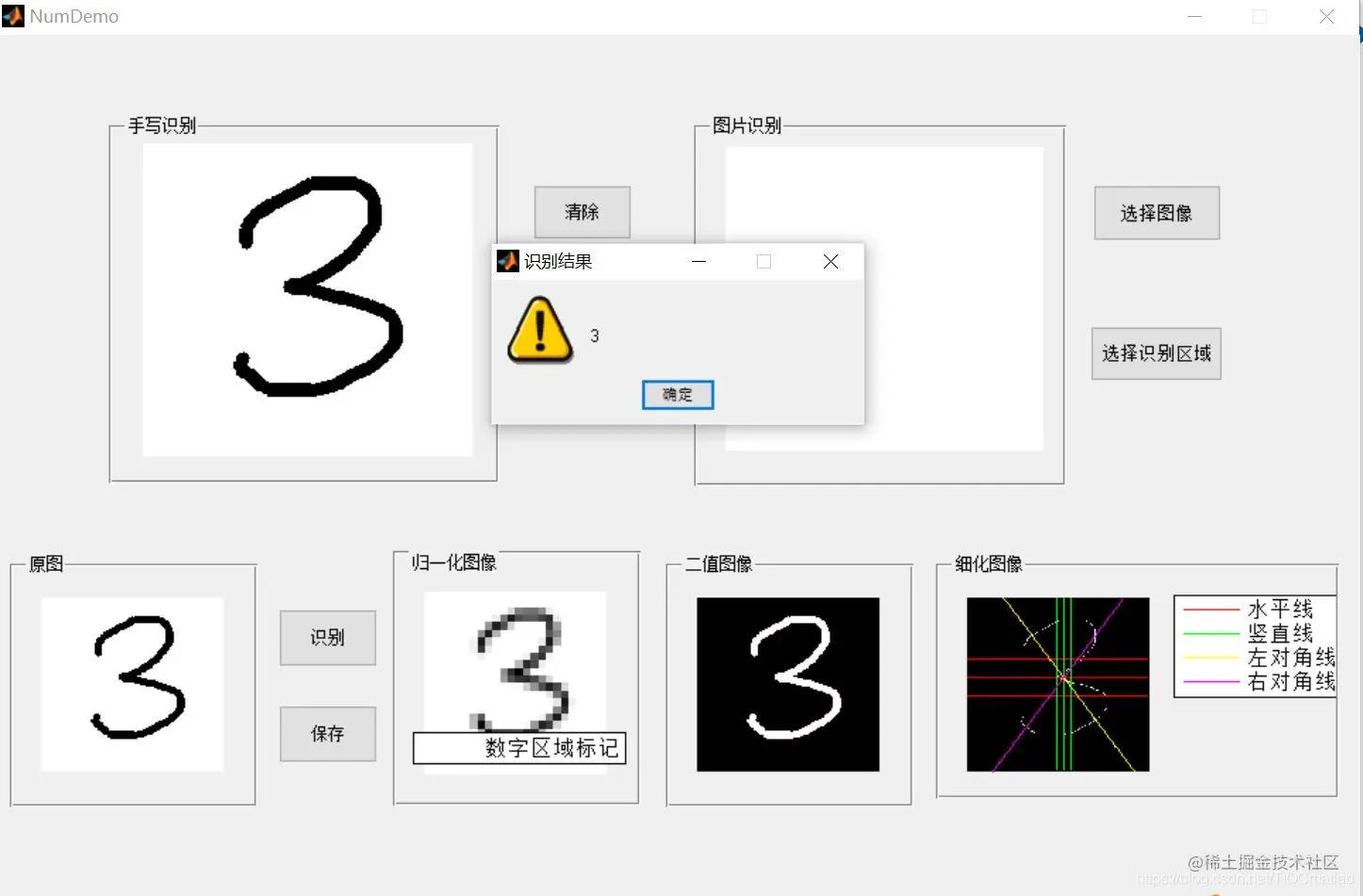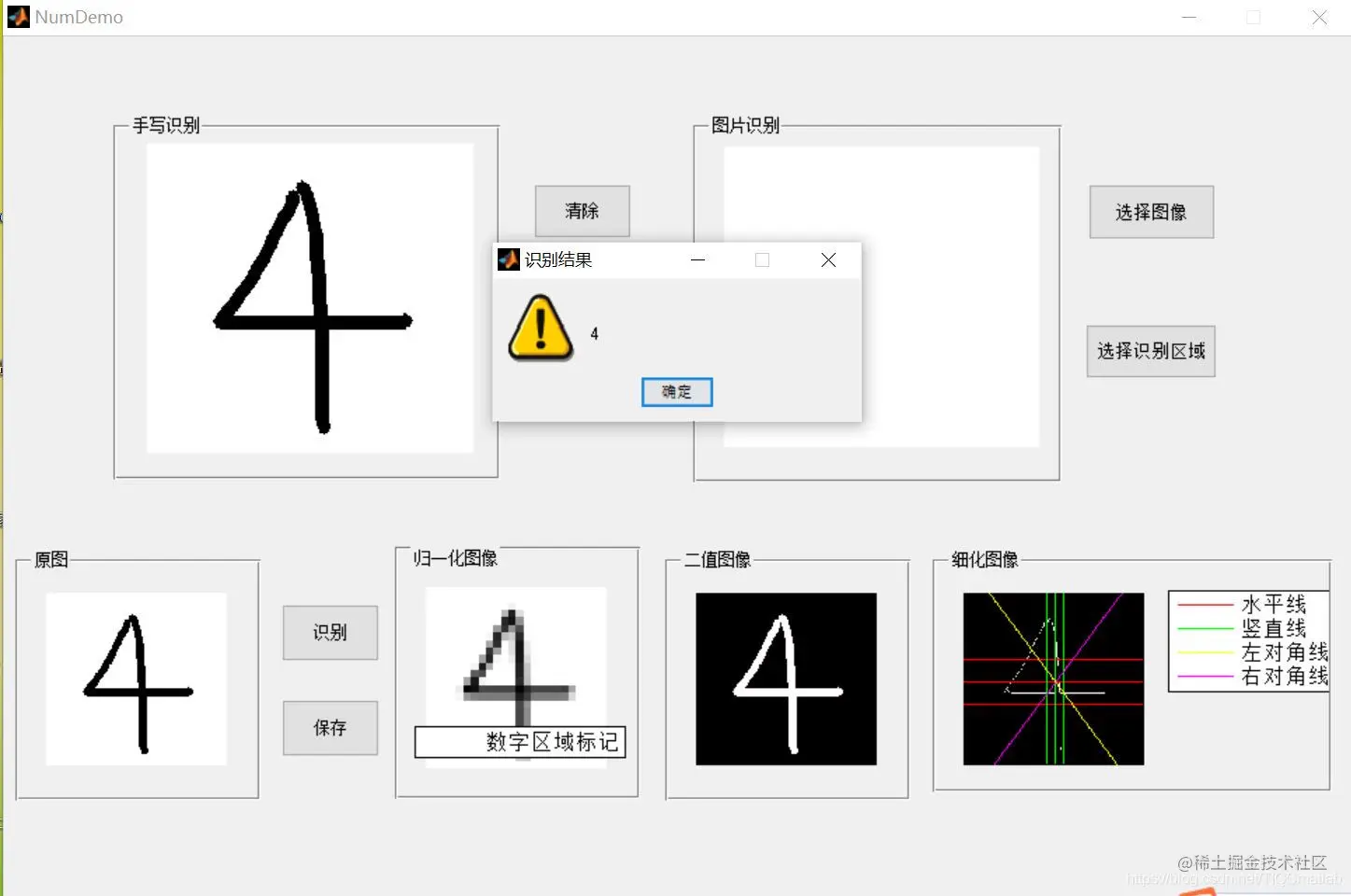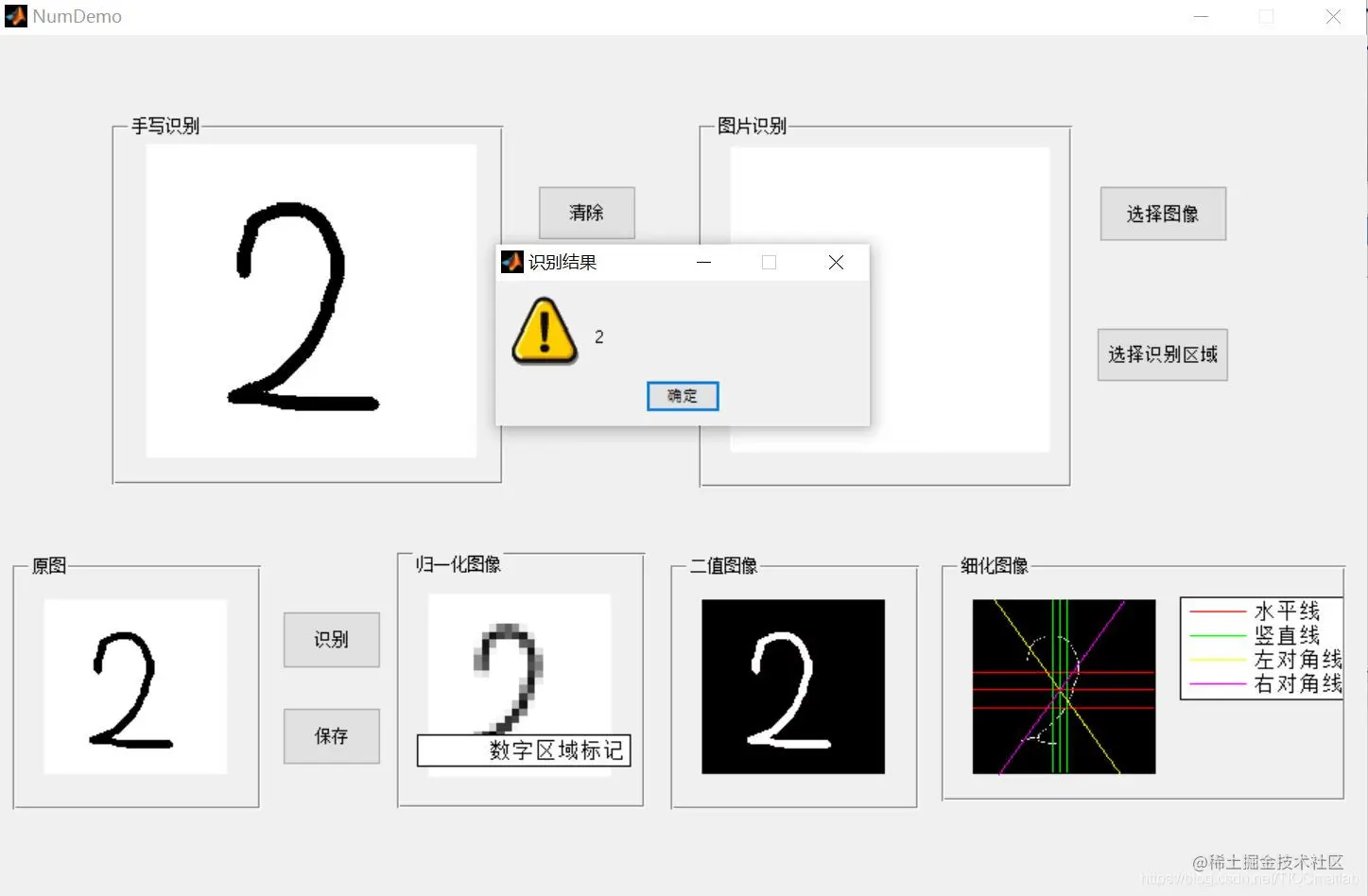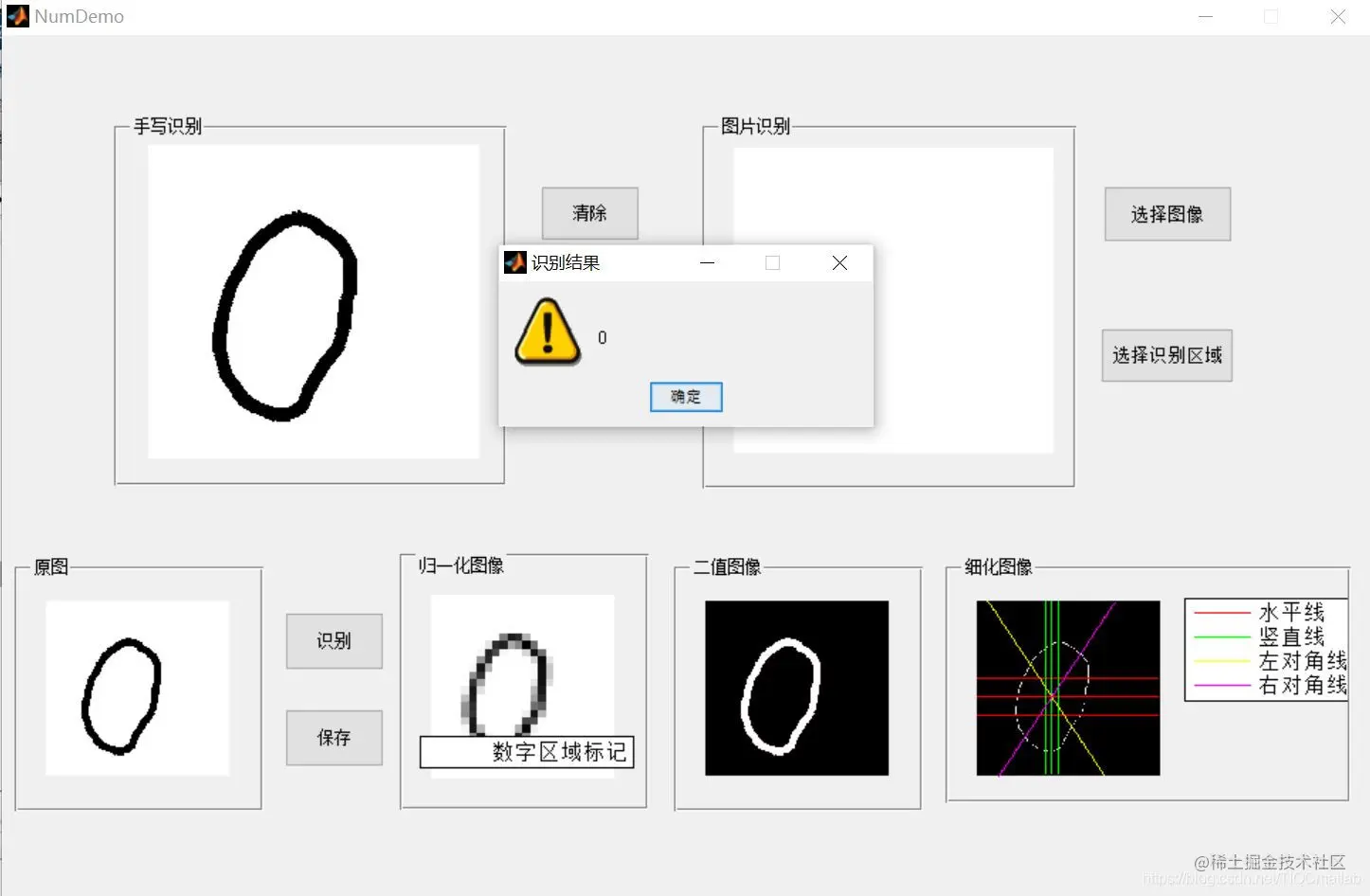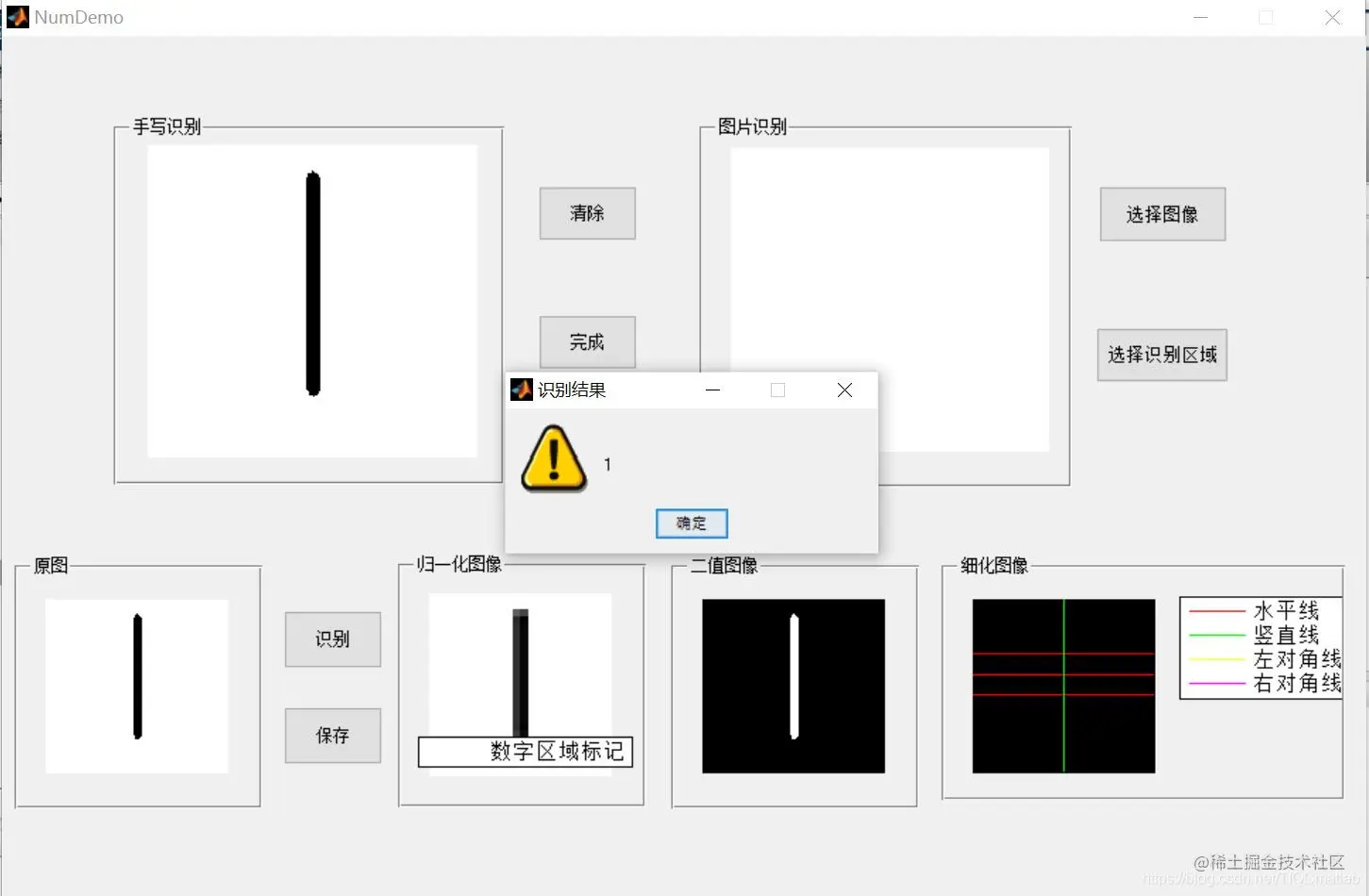## Four 、matlab Edition and references

1 matlab edition 2014a

2 reference  Cai Limei .MATLAB The image processing —— theory 、 Algorithm and example analysis [M]. tsinghua university press ,2020.  Yang Dan , Zhao Haibin , Long Zhe .MATLAB Detailed explanation of image processing examples [M]. tsinghua university press ,2013.  Zhou pin .MATLAB Image processing and graphical user interface design [M]. tsinghua university press ,2013.  Liu Chenglong . Master MATLAB The image processing [M]. tsinghua university press ,2015.